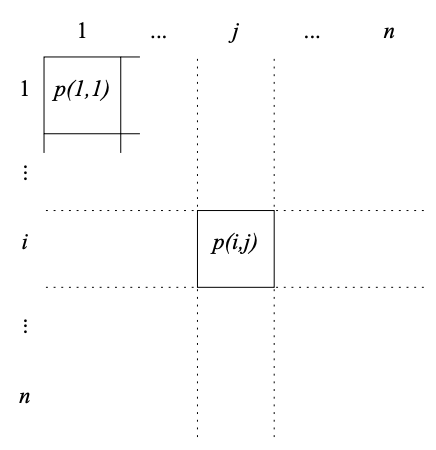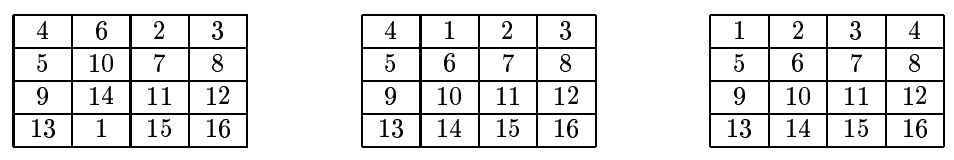시간 제한 메모리 제한 제출 정답 맞은 사람 정답 비율
1 초 128 MB 0 0 0 0.000%

## 문제

The king of Byteland has received a gift, a jigsaw puzzle. The puzzle consists of a board of size n×n. The field in the i-th row and j-th column (1 ≤ i, j ≤ n) has coordinates (i, j) and contains a piece with the number p(i, j), 1 ≤ p(i, j) ≤ n2. Each of the numbers 1 ... n2 appears on exactly one of the pieces.Coordinates of the fields

To solve the puzzle, you have to put the pieces in order, so that for each 1 ≤ i, j ≤ n the field (i, j) contains the piece with number j + (i - 1) × n.

The following moves are allowed to solve the puzzle:

• a cyclic shift of all pieces in a row a certain number of fields to the right,
• a cyclic shift of all pieces in a column a certain number of fields down.

The king of Byteland managed to solve his puzzle, but he is not sure if he would be able to solve it starting from a different initial configuration. Help him to solve this problem.

Write a program that:

• Reads from the standard input the description of the initial configurationof the puzzle.
• Finds out if the pieces on the board can be put in order using only the moves given above. If the solution is possible, the program should find the moves putting the pieces in order.
• Writes the result to the standard output.

## 입력

In the first line of the standard input there is one integer n the size of the board side (2 ≤ n ≤ 200). The following n lines contain the description of the initial configuration. The line i + 1 contains n integers p(i, 1), p(i, 2), ..., p(i, n)separated by single spaces.

## 출력

If there is no solution, the program should write to the standard output only one line containing only one word NO.

If a solution exists, the first line should contain one integer m the number of moves leading to the solution of the puzzle. The number of moves in your solution must not exceed 400 000. The following m lines should contain the descriptions of the moves, one move per line.

Each such line should consist of a letter R (for shifting a row to the right) or C (for shifting a column down), a space, and two integers: k and l separated by a space; 1 ≤ k ≤ n, 1 ≤ l ≤ n − 1. A line containing R k l describes a cyclic shift of the k-th row l fields to the right. Such a move leads to the following

board configuration: $p'(i,j) = \begin{cases} p(i,j+n-l) & \text{if } i = k \text { and }j \le l\\ p(i, j-l) & \text{if } i = k \text{ and } j > l \\ p(i, j)) & \text {if } i \ne k\end{cases}$ Similarly, a line containing C k l describes a cyclic shift of the k-th column filelds down.

If there are several possible solutions, your program should output anyon eof them.

## 예제 입력 1

4
4 6 2 3
5 10 7 8
9 14 11 12
13 1 15 16


## 예제 출력 1

2
C 2 1
R 1 3


## 힌트

The above sequence of moves gives the following sequence of configurations: